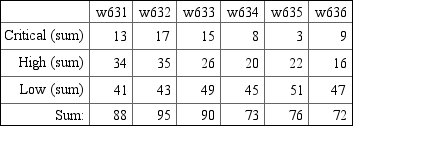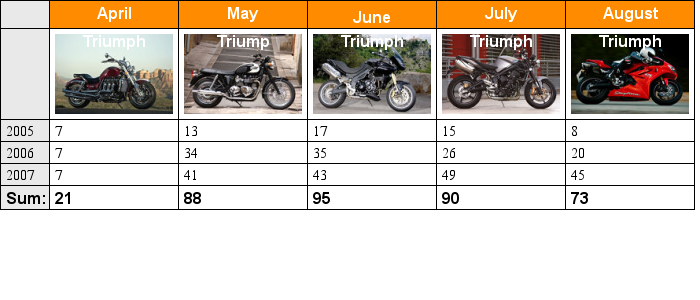## 19.5. Examples

### 19.5.1. Example 1

Table formatting, variant 1, right adjusted Times font

Figure 19.14. xx `(table_mex00.php)`### 19.5.2. Example 2

Table formatting, variant 1, using Times font for data and a sans serit font for headings together with removed grid lines and filled headers.

Figure 19.15. xx `(table_mex0.php)`### 19.5.3. Example 3

Table formatting, variant 2, using the same sans serit font for all information but with some grid lines.

Figure 19.16. xx `(table_mex1.php)`### 19.5.4. Example 4

Table formatting, variant 3, where some data is highlighted and we use a thicker border.

Figure 19.17. xx `(table_mex3.php)`### 19.5.5. Example 5

Show how the table can be turned 90 degrees

Figure 19.18. Vertical text `(table_vtext.php)`### 19.5.6. Example 6

The following example shows how to add images (icons) to a number of cells.

Figure 19.19. Adding an icon (image) in a table cell `(table_iconex1.php)`